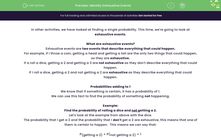# Identify Exhaustive Events

In this worksheet, students will practise identifying exhaustive events and look at the probability of an event not happening.Key stage:  KS 4

Year:  GCSE

GCSE Subjects:   Maths

GCSE Boards:   AQA, Eduqas, Pearson Edexcel, OCR,

Curriculum topic:   Probability

Curriculum subtopic:   Probability Combined Events and Probability Diagrams

Difficulty level:#### Worksheet Overview

In other activities, we have looked at finding a single probability. This time, we're going to look at exhaustive events.

What are exhaustive events?

Exhaustive events are two events that describe everything that could happen.

For example, if I throw a coin, getting a head and getting a tail are the only two things that could happen, so they are exhaustive.

If a roll a dice, getting a 2 and getting a 3 are not exhaustive as they don't describe everything that could happen.

If I roll a dice, getting a 2 and not getting a 2 are exhaustive as they describe everything that could happen.

We know that if something is certain, it has a probability of 1.

We can use this fact to find the probability of something not happening.

Example:

Find the probability of rolling a dice and not getting a 2.

Let's look at the example from above with the dice.

The probability that I get a 2 and the probability that I don't get a 2 are exhaustive, this means that one of them is certain to happen.  This means we can say that:

P(getting a 2) + P(not getting a 2) = 1

If we rearrange this...

P(not getting a 2) = 1 - P(getting a 2).

This means that if we know the probability of something happening, we can find the probability of it not happening.

P(not getting a 2) = 1 - 1/6 = 5/6

Time for some questions now.

### What is EdPlace?

We're your National Curriculum aligned online education content provider helping each child succeed in English, maths and science from year 1 to GCSE. With an EdPlace account you’ll be able to track and measure progress, helping each child achieve their best. We build confidence and attainment by personalising each child’s learning at a level that suits them.

Get started